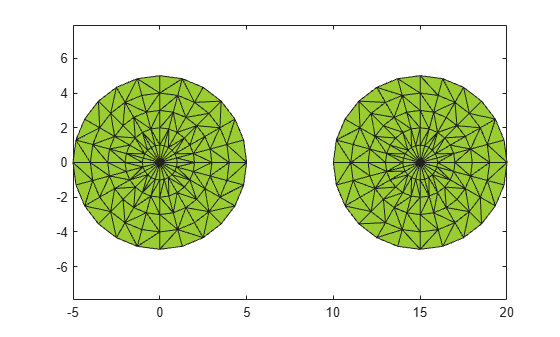Documentation

# numRegions

Number of regions in alpha shape

## Syntax

``N = numRegions(shp)``

## Description

example

````N = numRegions(shp)` returns the number of distinct regions that make up the alpha shape. For an alpha radius of `Inf`, the alpha shape is the convex hull, and the number of regions is one. As the value of the alpha radius decreases the shape can break into separate regions, depending on the point set.```

## Examples

collapse all

Create and plot a set of 2-D points.

```th = (pi/12:pi/12:2*pi)'; x1 = [reshape(cos(th)*(1:5), numel(cos(th)*(1:5)),1); 0]; y1 = [reshape(sin(th)*(1:5), numel(sin(th)*(1:5)),1); 0]; x = [x1; x1+15;]; y = [y1; y1]; plot(x,y,'.') axis equal```Create an alpha shape using an alpha radius of 7 and query the number of distinct regions in the shape.

```shp = alphaShape(x,y,7); nregions = numRegions(shp)```
```nregions = 1 ```

Use a smaller alpha radius of 2.5 to better capture the boundary and then retrieve the new number of distinct regions.

```shp.Alpha = 2.5; nregions = numRegions(shp)```
```nregions = 2 ```

Plot the alpha shape to check the boundary quality.

`plot(shp)`## Input Arguments

collapse all

Alpha shape, specified as an `alphaShape` object. For more information, see `alphaShape`.

Example: `shp = alphaShape(x,y)` creates a 2-D `alphaShape` object from the `(x,y)` point coordinates.